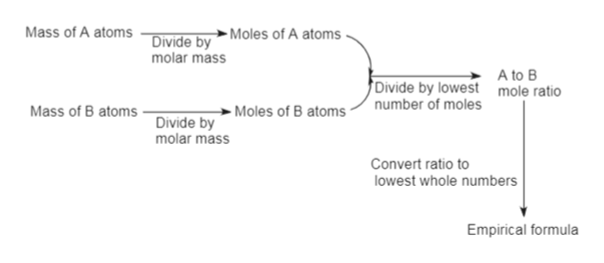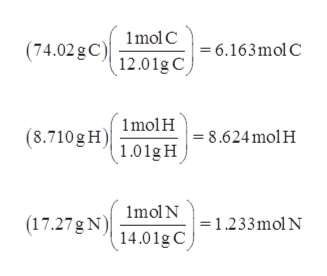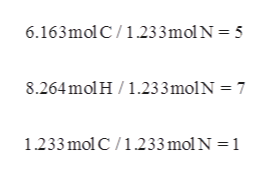# provide an example of how to calculate empirical formula and then how to find molecular formula from this

Question
12 views

provide an example of how to calculate empirical formula and then how to find molecular formula from this

check_circle

Step 1

In the determination of molecular formula, first step is the determination of its empirical formula.help_outlineImage TranscriptioncloseMass of A atomsDivide by Moles of A atoms molar mass A to B mole ratio Divide by lowest number of moles Mass of B atoms Moles of B atoms Divide by molar mass Convert ratio to lowest whole numbers Empirical formula fullscreen
Step 2

Take Nicotine as an example, and assume it contains 74.02 % C, 8.710 % H, and 17.27% N.  Consider, 40.57 g of Nicotine consists of 0.25 mol Nicotine.

First, calculate the moles of each element,help_outlineImage Transcriptionclose(74.02gCImol C6.163mol C 12.01g C (8.710 g HImolH8.624molH 1.01g H (17.27gN mol N1.233mol Nn 14.01g C fullscreen
Step 3

Calculate the molar ratio...help_outlineImage Transcriptionclose6.163mol C/1.233mol N = 5 8.264molH 1.233molN = 7 1.233 mol C/1.233 molN =1 fullscreen

### Want to see the full answer?

See Solution

#### Want to see this answer and more?

Solutions are written by subject experts who are available 24/7. Questions are typically answered within 1 hour.*

See Solution
*Response times may vary by subject and question.
Tagged in

### General Chemistry